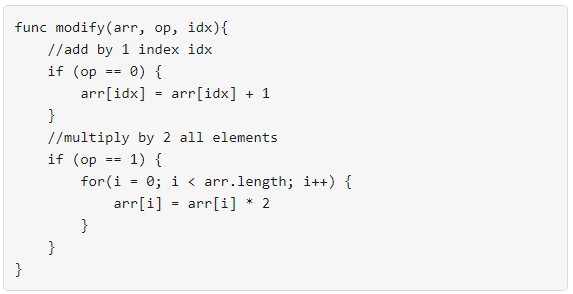Formatted question description: https://leetcode.ca/all/1558.html

# 1558. Minimum Numbers of Function Calls to Make Target Array (Medium)Your task is to form an integer array nums from an initial array of zeros arr that is the same size as nums.

Return the minimum number of function calls to make nums from arr.

The answer is guaranteed to fit in a 32-bit signed integer.

Example 1:

Input: nums = [1,5]
Output: 5
Explanation: Increment by 1 (second element): [0, 0] to get [0, 1] (1 operation).
Double all the elements: [0, 1] -> [0, 2] -> [0, 4] (2 operations).
Increment by 1 (both elements)  [0, 4] -> [1, 4] -> [1, 5] (2 operations).
Total of operations: 1 + 2 + 2 = 5.


Example 2:

Input: nums = [2,2]
Output: 3
Explanation: Increment by 1 (both elements) [0, 0] -> [0, 1] -> [1, 1] (2 operations).
Double all the elements: [1, 1] -> [2, 2] (1 operation).
Total of operations: 2 + 1 = 3.


Example 3:

Input: nums = [4,2,5]
Output: 6
Explanation: (initial)[0,0,0] -> [1,0,0] -> [1,0,1] -> [2,0,2] -> [2,1,2] -> [4,2,4] -> [4,2,5](nums).


Example 4:

Input: nums = [3,2,2,4]
Output: 7


Example 5:

Input: nums = [2,4,8,16]
Output: 8


Constraints:

• 1 <= nums.length <= 10^5
• 0 <= nums[i] <= 10^9

Related Topics:
Greedy

## Solution 1.

For each number in A, we can compute the number of operations needed to modify it to 0. We count add and mul separately.

In the final result, we need to sum the count of all the add operations together because we have to do them independently for each A[i]. But for the mul operation, we just need to take the maximum one.

// OJ: https://leetcode.com/problems/minimum-numbers-of-function-calls-to-make-target-array/

// Time: O(Nlog(max(A)))
// Space: O(1)
class Solution {
public:
int minOperations(vector<int>& A) {
int add = 0, mul = 0;
for (int n : A) {
int m = 0;
while (n) {
if (n % 2) ++add, --n;
else ++m, n /= 2;
}
mul = max(mul, m);
}
return add + mul;
}
};


Java

class Solution {
public int minOperations(int[] nums) {
int operations = 0;
boolean flag = true;
int length = nums.length;
while (flag) {
flag = false;
for (int i = 0; i < length; i++) {
if (nums[i] % 2 != 0) {
nums[i]--;
operations++;
}
if (nums[i] > 0) {
flag = true;
nums[i] /= 2;
}
}
if (flag)
operations++;
}
return operations;
}
}